Rationalizing denominatorsCustom SearchRATIONALIZING DENOMINATORS.- The product of the sum and difference of two numbers is useful in rationalizing a denominator that is a binomial. For example, in a fraction such as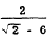the denominator can be altered so that no radical terms appear in it. (This process is called rationalizing.) The denominator must be multiplied by+ 6, which is called the conjugate of- 6. Since the value of the original fraction would be changed if we multiplied only the denominator, our multiplier must be applied to both the numerator and the denominator. Multiplying the original fraction by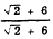is, in effect, the same as multiplying it by 1. The result of rationalizing the denominator of this fraction is as follows:MENTAL MULTIPLICATION.-The product of the sum and difference can be utilized to mentally multiply two numbers that differ from a multiple of 10 by the same amount, one greater and the other less. For example, 67 is 3 less than 70 while 73 is 3 more than 70. The product of 67 and 73 is then found as follows: 67(73) = (70 - 3)(70 + 3) = 702 - 32 = 4,900 - 9 = 4,891 Square of Sum or Difference The square of the SUM of two numbers is equal to the square of the first number plus twice the product of the numbers plus the square of the second number. The square of the DIFFERENCE of the same two numbers has the same form, except that the sign of the middle term is negative. These results are evident from multiplication. When x and y represent the two numbers, we obtainApplying this rule to the squares of the binomials 3a + 2b and 3a - 2b, we have the following two cases: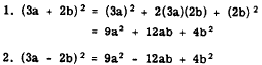The square of the sum or difference of two numbers is applicable to squaring a binomial that contains one or two irrational terms, as in the following examples :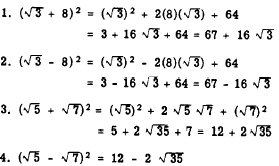The square of the sum or difference of two numbers can be applied to the process of mentally squaring certain numbers. For example, 822 can be expressed as (80 + 2)2 while 672 can be expressed as (70 - 3)2. We find that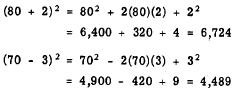Binomials Having a Common Term The binomials x + 2 and x - 3 have a common term, x. They have two unlike terms, +2 and -3. The product of these binomials isInspection of this product shows that it is obtained by squaring the common term, adding the sum of the unlike terms multiplied by the common term, and finally adding the product of the unlike terms. Apply this rule to the product of 3y - 5 and 3y + 4. The common term is 3y; its square is 9y2. The sum of the unlike terms is -5 + 4 = - 1; the sum of the unlike terms multiplied by the common term is -3y; and the product of the unlike terms is -5(4) = -20. The product of the two binomials is (3Y - 5)(3y + 4) = 9y2 - 3y - 20 The product of two binomials having a common term is applicable to the multiplication of numbers like+ 7 and- 2 which contain irrational terms. For example,Practice problems. In problems 1 through 4, multiply and combine terms. In 5 through 8, simplify by using special products.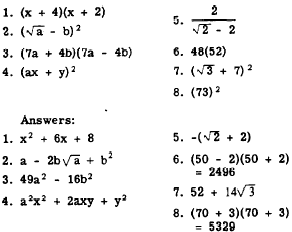Integrated Publishing, Inc. - A (SDVOSB) Service Disabled Veteran Owned Small Business